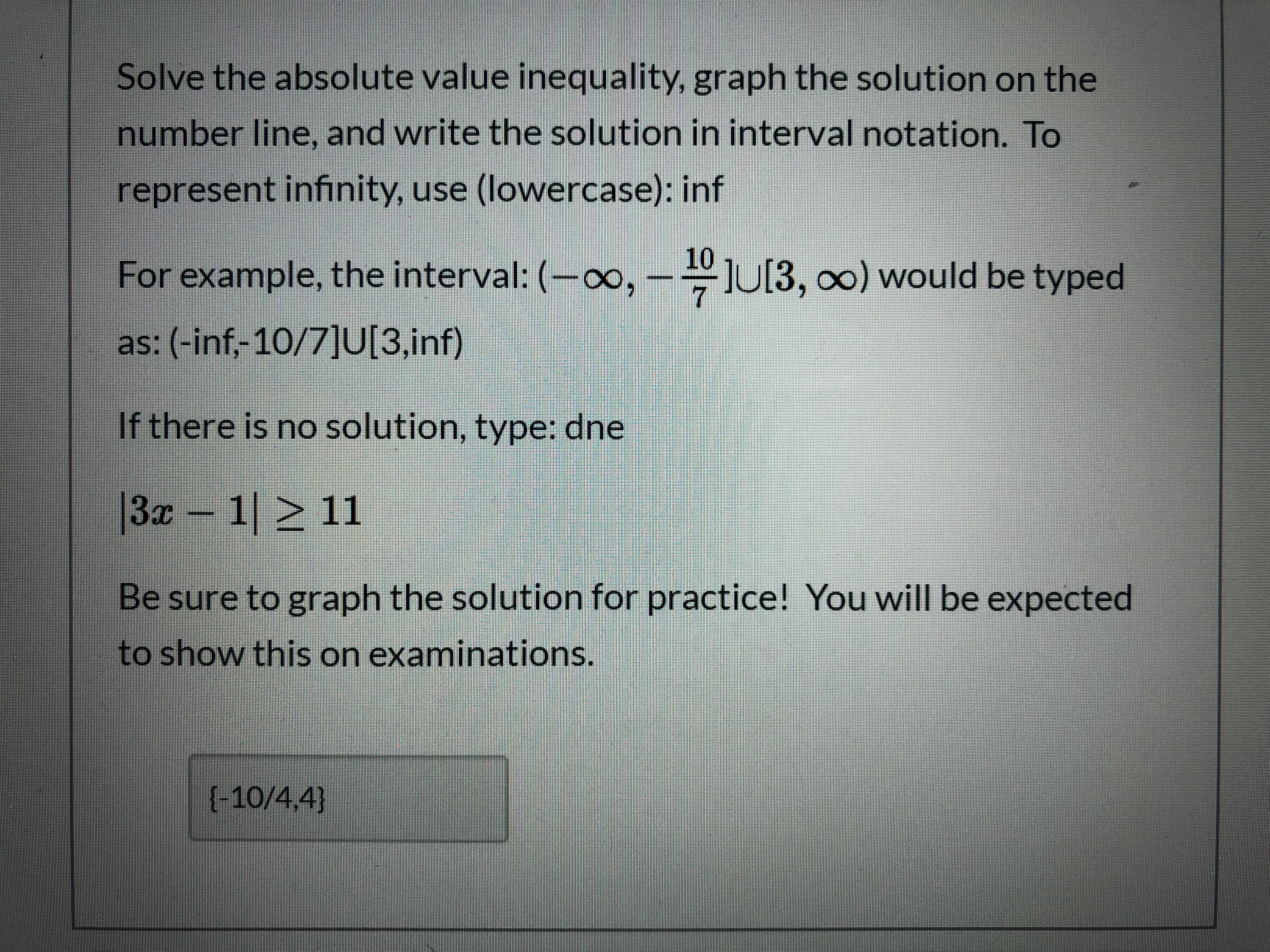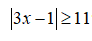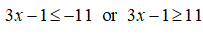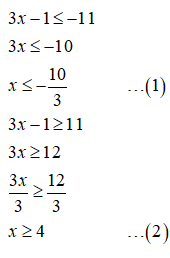# Solve the absolute value inequality, graph the solution on thenumber line, and write the solution in interval notation. Torepresent infinity, use (lowercase): inf10For example, the interval: (-∞, –-U[3, o0) would be typedas: (-inf,-10/7]U[3,inf)If there is no solution, type: dne|3z 1 > 11Be sure to graph the solution for practice! You will be expectedto show this on examinations.{-10/4,4}

Question
1 views

I don't understand how to solve this can you helphelp_outlineImage TranscriptioncloseSolve the absolute value inequality, graph the solution on the number line, and write the solution in interval notation. To represent infinity, use (lowercase): inf 10 For example, the interval: (-∞, –-U[3, o0) would be typed as: (-inf,-10/7]U[3,inf) If there is no solution, type: dne |3z 1 > 11 Be sure to graph the solution for practice! You will be expected to show this on examinations. {-10/4,4} fullscreen
check_circle

Step 1

The given inequality is,Step 2

Applying absolute rule on the above inequality gives,Step 3

Now obtain the interval for x as follows....

### Want to see the full answer?

See Solution

#### Want to see this answer and more?

Solutions are written by subject experts who are available 24/7. Questions are typically answered within 1 hour.*

See Solution
*Response times may vary by subject and question.
Tagged in

### Algebra